# Recursive function

(diff) ← Older revision | Latest revision (diff) | Newer revision → (diff)

partial recursive function

One of the mathematical precizations of the intuitive concept of a computable function, defined as follows. One examines functions given on the natural numbers and with natural values. The functions are assumed to be partial, i.e. they are not defined, generally speaking, for all values of the arguments. The following functions are called basic:,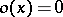,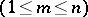. One says that an-place functionis obtained from an-place functionand-place functions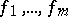with the aid of composition if for allthe following equality holds: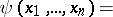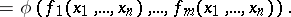One says that an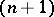-place functionis obtained from an-place functionand the-place functionby means of primitive recursion if for any values of, the following equalities hold: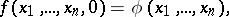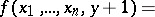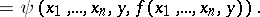One says that an-place functionis obtained from the-place functionwith the aid of a minimization operator, or least-number operator, if for any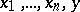the condition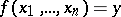holds if and only if the values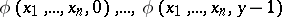are defined and are not equal to 0, while. A partial functionis called recursive if it can be obtained from the basic functions by means of a finite number of applications of composition, primitive recursion and minimization operators.

In other words,is a recursive function if there is a finite sequence of partial functionssuch that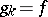, and each function in this sequence is either basic or is obtained from the previous functions by means of composition, primitive recursion or minimization. With the method of arithmetization it is possible to obtain an enumeration of all such descriptions of a recursive function, and in fact an algorithm can be given which computes a one-to-one surjective encoding between descriptions of recursive functions and the natural numbers. The recursive function being defined by this description is usually denoted by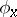, andis called its Gödel number.

An everywhere-defined recursive function is called general recursive. There are recursive functions that cannot be extended to general recursive functions.

For any recursive function one can give an algorithm for calculating its values, i.e. all recursive functions are in essence computable. A widespread hypothesis, known as Church's thesis, consists in the fact that every computable function is recursive. This thesis is confirmed by a number of facts. Thus, all concrete functions studied in mathematics and recognizable as computable in the intuitive sense of the word, proved to be recursive. The concept of a recursive function turns out to coincide in extent with other mathematical precizations of the concept of a computable function (e.g. with the concept of a function computable on a Turing machine, computable using a Markov normal algorithm, etc.).

There are several definitions of the class of all recursive functions by way of initial functions and generating operators. In particular, every recursive function can be obtained from the functions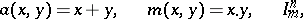andusing a finite number of composition and minimization operators.

How to Cite This Entry:
Recursive function. Encyclopedia of Mathematics. URL: http://encyclopediaofmath.org/index.php?title=Recursive_function&oldid=13434
This article was adapted from an original article by V.E. Plisko (originator), which appeared in Encyclopedia of Mathematics - ISBN 1402006098. See original article